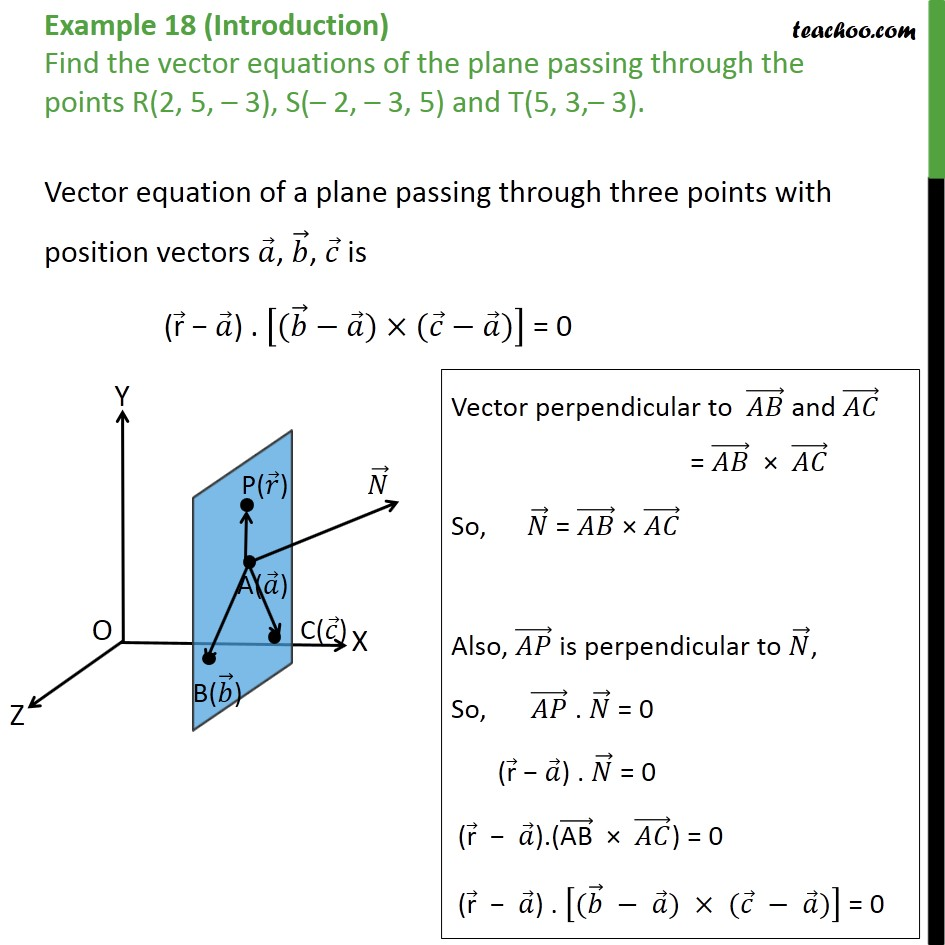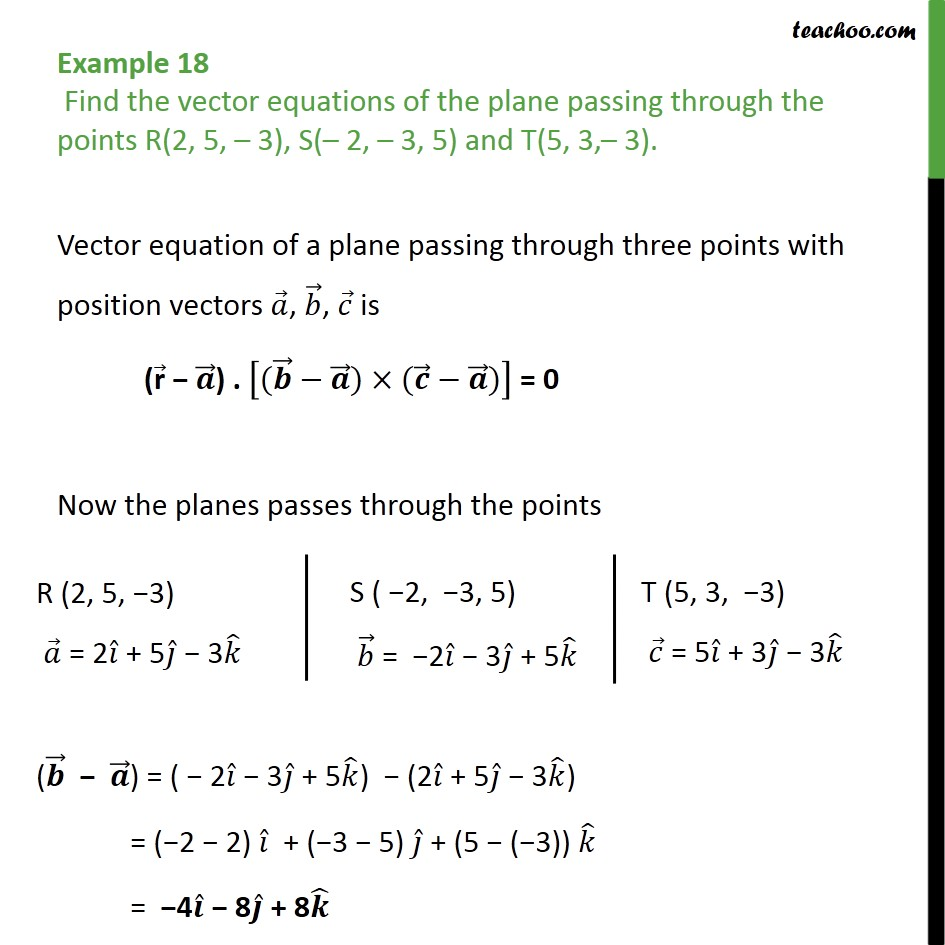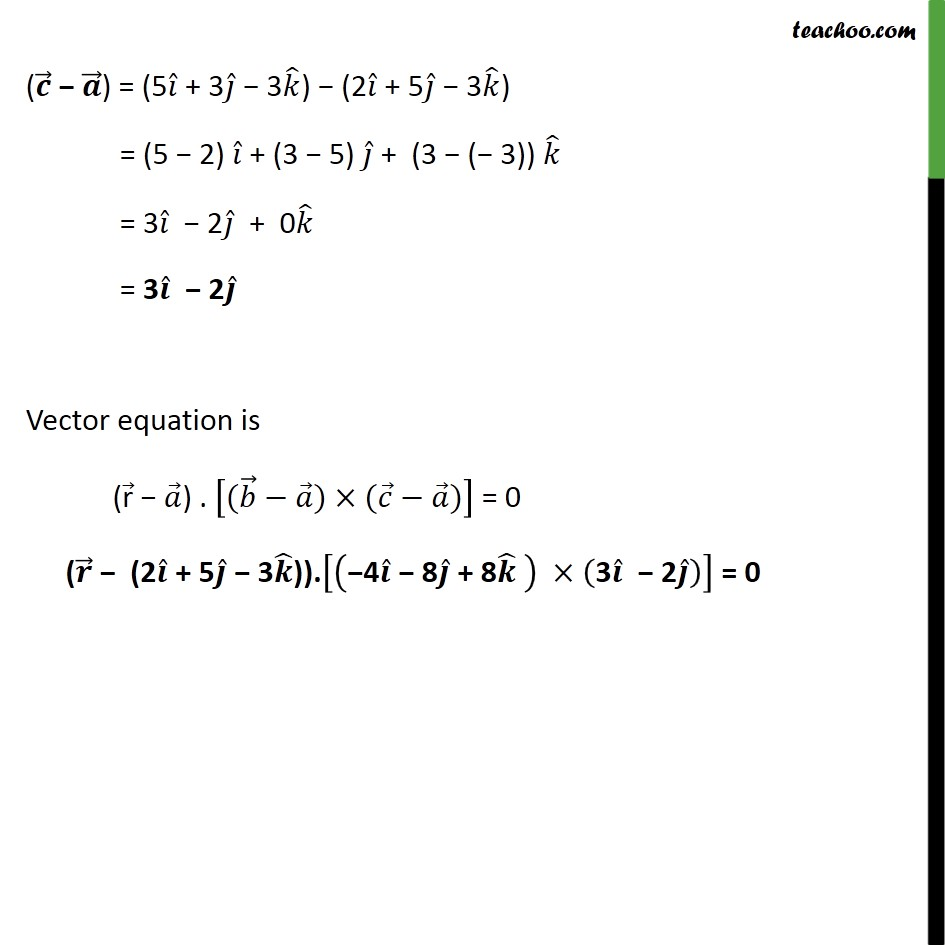Examples

Chapter 11 Class 12 Three Dimensional Geometry
Serial order wiseLearn in your speed, with individual attention - Teachoo Maths 1-on-1 Class

### Transcript

Question 8(Introduction) Find the vector equations of the plane passing through the points R(2, 5, 3), S( 2, 3, 5) and T(5, 3, 3). Vector equation of a plane passing through three points with position vectors , , is ( r ) . ( ) ( ) = 0 Example 18 Find the vector equations of the plane passing through the points R(2, 5, 3), S( 2, 3, 5) and T(5, 3, 3). Vector equation of a plane passing through three points with position vectors , , is ( r ) . ( ) ( ) = 0 Now the planes passes through the points ( ) = (5 + 3 3 ) (2 + 5 3 ) = (5 2) + (3 5) + (3 ( 3)) = 3 2 + 0 = 3 2 Vector equation is ( r ) . ( ) ( ) = 0 ( (2 + 5 3 )). 4 8 + 8 3 2 = 0